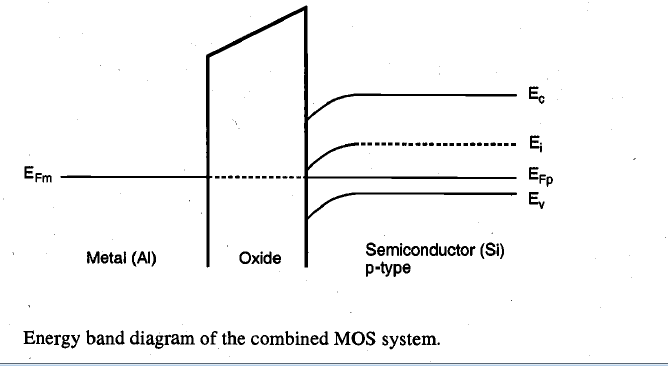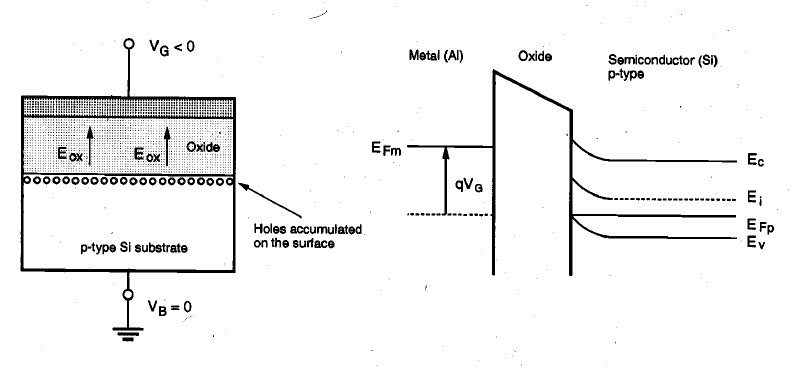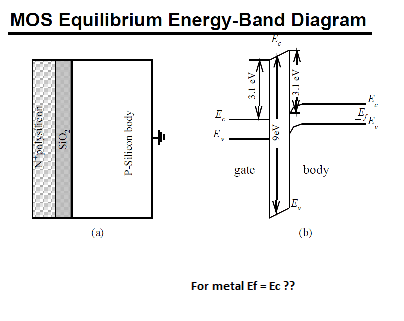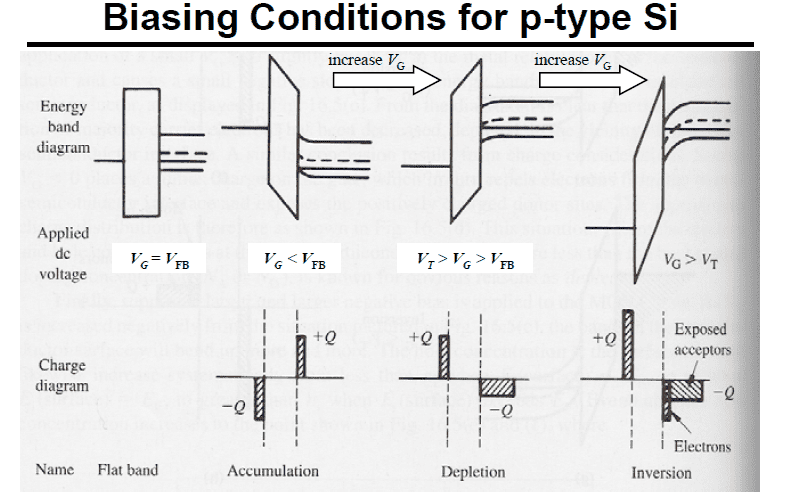# Why does Efm shift in Mosfets

• A
Hi,
I'm studying MOSFET and the book says that when N-channel mosfet has negative or positive gate voltage, the energy band diagram will shift. I don't understand why Efm shifts.Now when Vg is negative the Efm shifts upwards, Why? And when later when Vf is positive the Efm also shifts again? I don't understand this. Shouldn't Efm and Efp be at same level?#### Attachments

Lord Jestocost
Gold Member
The Fermi level (or electrochemical potential of the electrons) is only constant throughout a MOS device when thermal equilibrium is reached and no external bias is applied to the device..

•jaus tail
Thanks for the reply. Shouldn't Efm and Efp be at same level. At junction of PN diode, we shift bands after aligning the Ef of both doped regions together.

Lord Jestocost
Gold Member
•jaus tail
Thanks. This helped clear some things up. Just to be sure. Let's assume Vfb = 0 for now. initially mosfet fermi and Si fermi are at same level.I got the above pic from the pdf link you gave.
Now if I apply positive Gate potential, then the positive terminal will attract electrons from Gate (metal). So the surface junction between Metal and Oxide will be devoid of electrons and so fermi level of metal will shift downwards.
Likewise the positive terminal will attract electrons from P-silicon body and fermi level of Semiconductor shifts up toward Ec at surface. Thus we bend Ec and Ev of Semiconductor so that Ec is closer to Ef at junction.

This pic helped a lot:But I don't understand How Vfb helps. I assumed Vfb = 0 and was able to figure out how bands shift. But can you elaborate as to how Vfb comes in the picture?
When Vg is less than Vfb as in accumulation case, is Vg also less than zero? That would explain why negative Q accumulates at junction. Cause negative Vg repels electrons to junction.

#### Attachments

Lord Jestocost
Gold Member
From “Physics and Technology of Semiconductor Devices” by Andrew S. Grove:

The electron energies at the Fermi level in the metal and in the semiconductor of an MOS structure will, in general, be different. Such an energy difference is usually expressed as a difference in work functions, which is the energy required to remove an electron from the Fermi level in a given material to vacuum. When the metal of an MOS structure is shorted to the semiconductor, electrons will flow from the metal to the semiconductor or vice versa until a potential will be built up between the two which will counterbalance the difference in work functions. When equilibrium is reached, the Fermi level in the metal is lined up with the Fermi level in the semiconductor. Therefore, there will be an electrostatic potential variation from one region to the other, as illustrated in….

Thus, when there is a difference in work function between the metal and the semiconductor, one has band bending within the semiconductor in the equilibrium case with gate voltage VG = 0. You can now apply just enough gate voltage VG to counterbalance the work function difference and to maintain a flat band condition in the semiconductor. The gate voltage required to bring about the flat band condition is called the flat band voltage VFB. VFB is - so to speak - the reference point for the gate voltage VG for further considerations.

Last edited:
•jaus tail